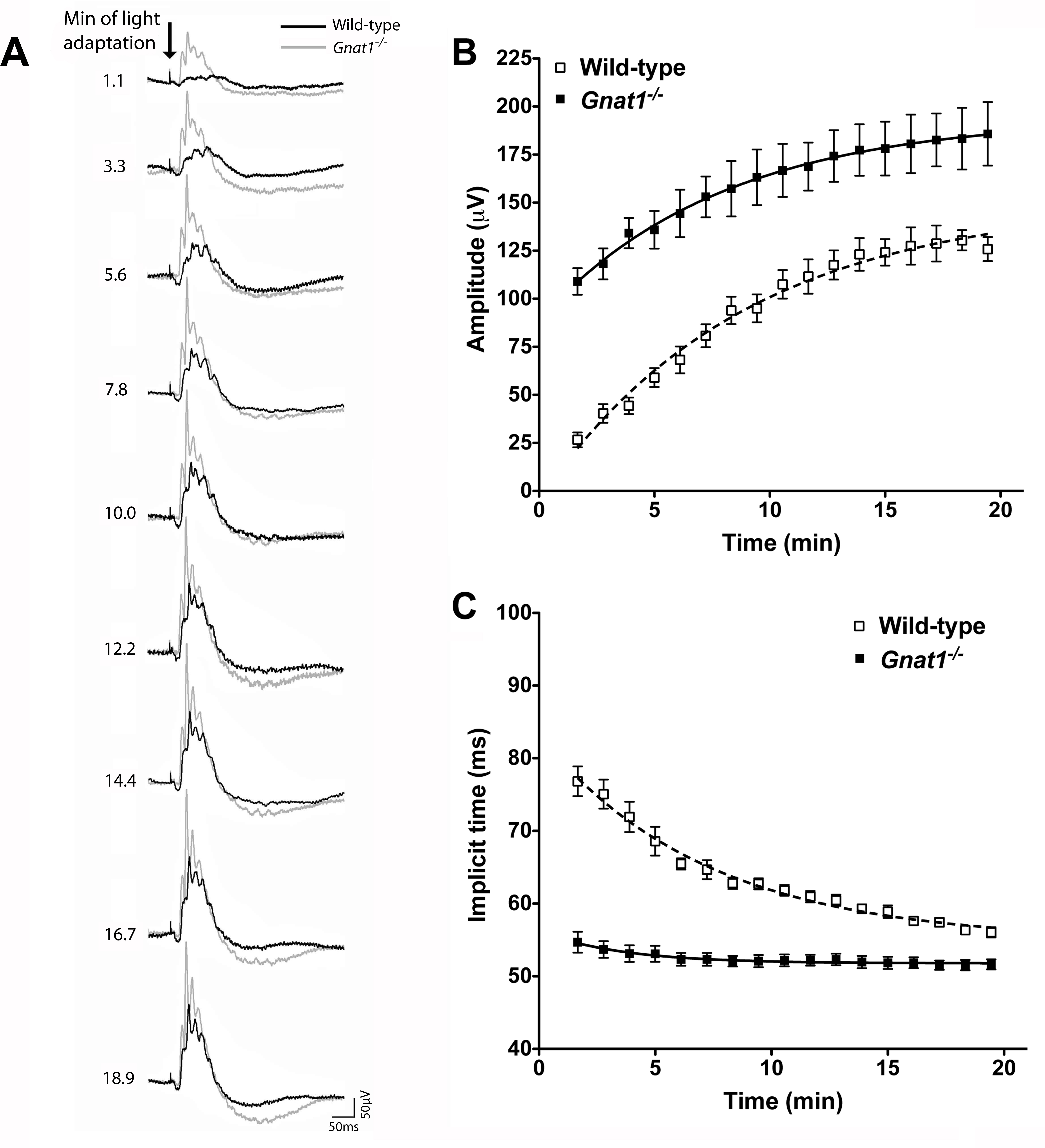Figure 2 of Cameron, Mol Vis 2009; 15:2209-2216.

Figure 2. Comparison of cone ERG traces from Gnat1−/− and wild-type mice over the course of light adaptation. A shows representative ERG traces from Gnat1−/− (gray trace) and wild-type (black trace) mice recorded at CT6 in response to a bright white flash superimposed on a rod saturating background. At the beginning of light adaptation (top), b-waves were much more noticeable in Gnat1−/− animals than in wild-types. The magnitude of this difference was reduced as light adaptation proceeded. The arrow in A depicts flash onset, scale bar=50 ms (x-axis), 50 μV (y-axis) and numbers to left correspond to the time exposed to the rod saturating background in minutes. B shows quantification of b-wave amplitude against time spent exposed to the adapting light in Gnat1−/− (closed squares) compared to wild-type (open squares). Gnat1−/− mice show significantly larger responses than wild-types (F test curve fit comparison p<0.0001). C shows that b-wave implicit time (IT) was much reduced in Gnat1−/− mice compared to wild-types across all time points (F test curve fit comparison p<0.0001). Data in B and C is shown as mean±SEM. Replicates for B and C are: n=10 for wild-types, n=8 for Gnat1−/−. All data mean±SEM; n=9 for wild-types, n=8 for Gnat1−/−. The estimated parameters for curve fit for the curves in B (wild-type) are, amplitude: Y0=-5.061 a=149, k=0.124, implicit time: Y0=83.39, a=54.94, k=0.143. The curve fit for C (Gnat1−/−) estimated parameters are, amplitude: Y0=88.72, a=194.4, k=0.127, implicit time: Y0=56.23, a=51.78, k=0.283.